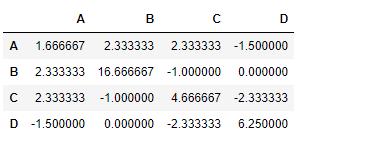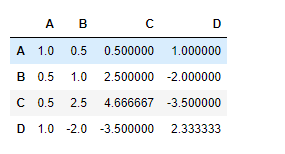# Python | Pandas dataframe.cov()

Python is a great language for doing data analysis, primarily because of the fantastic ecosystem of data-centric python packages. Pandas is one of those packages and makes importing and analyzing data much easier.

Pandas` dataframe.cov()` is used to compute pairwise covariance of columns.
If some of the cells in a column contain `NaN` value, then it is ignored.

Syntax: DataFrame.cov(min_periods=None)

Parameters:
min_periods : Minimum number of observations required per pair of columns to have a valid result.

Returns: y : DataFrame

Example #1: Use `cov()` function to find the covariance between the columns of the dataframe.

Note : Any non-numeric columns will be ignored.

 `# importing pandas as pd ` `import` `pandas as pd ` ` `  `# Creating the dataframe ` `df ``=` `pd.DataFrame({``"A"``:[``5``, ``3``, ``6``, ``4``],  ` `                   ``"B"``:[``11``, ``2``, ``4``, ``3``], ` `                   ``"C"``:[``4``, ``3``, ``8``, ``5``], ` `                   ``"D"``:[``5``, ``4``, ``2``, ``8``]}) ` ` `  `# Print the dataframe ` `df `

Output :Now find the covariance among the columns of the data frame

 `# To find the covariance  ` `df.cov() `

Output :Example #2: Use `cov()` function to find the covariance between the columns of the dataframe which are having `NaN` value.

 `# importing pandas as pd ` `import` `pandas as pd ` ` `  `# Creating the dataframe ` `df ``=` `pd.DataFrame({``"A"``:[``5``, ``3``, ``None``, ``4``], ` `                   ``"B"``:[``None``, ``2``, ``4``, ``3``], ` `                   ``"C"``:[``4``, ``3``, ``8``, ``5``],  ` `                   ``"D"``:[``5``, ``4``, ``2``, ``None``]}) ` ` `  `# To find the covariance  ` `df.cov() `

Output :My Personal Notes arrow_drop_upCheck out this Author's contributed articles.

If you like GeeksforGeeks and would like to contribute, you can also write an article using contribute.geeksforgeeks.org or mail your article to contribute@geeksforgeeks.org. See your article appearing on the GeeksforGeeks main page and help other Geeks.

Please Improve this article if you find anything incorrect by clicking on the "Improve Article" button below.

Article Tags :

Be the First to upvote.

Please write to us at contribute@geeksforgeeks.org to report any issue with the above content.